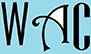## Geometry Question for Title 1 Schools: Triangle Height Question?

10) What is the height of a triangle with an area of 66 and base of 12?

Area of triangle = 1/2 x base x height “h”

66 = 1/2 x 12 x h

66 = 6 x h

you have to make “h” subject of formula by moving “6” to the other side.  When you go to the other side, addition becomes subtraction, multiplication becomes division, and vice versa.

66/6 = h (6 multiplied h at the other side)

11 = h

so the height of the triangle = 11